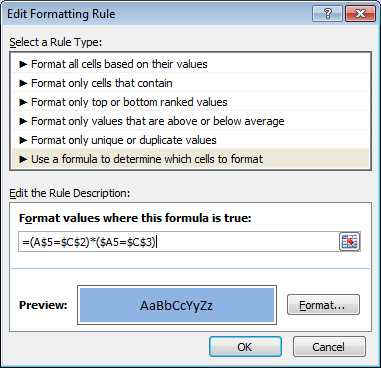Author: Oscar Cronquist Article last updated on March 23, 2019

In this article, I will demonstrate how to search a table using conditional formatting. The criteria highlight matching column and rows.

I am also going to explain how to create the highlighting and the conditional formatting formulas behind.

### Setting up Conditional Formatting

1. Select cell range A5:D25.
2. Go to tab "Home".
3. Click the "Conditional formatting" button.
4. Click "New Rule..".
5. Click "Use a formula to determine which cells to format".6. Type conditional formatting formula.
7. Click "Format..." button.
8. Select a color.
9. Click OK.
10. Click OK.

Repeat the above steps and create new conditional formatting rules with these formulas and colors:

and

### Explaining conditional formatting formulas

It is important to understand how relative and absolute cell references work when it comes to conditional formatting. Remember that cell range A5:D25 was selected before you created the conditional formatting rules, that will be the range the conditional formatting is applied to.

The first conditional formatting formula is:

=(A\$5=\$C\$2)*(\$A5=\$C\$3)

There are two logical expressions in this formula

• (A\$5=\$C\$2)
• (\$A5=\$C\$3)

They return TRUE if the condition is met or FALSE if not. The asterisk multiplies the boolean values and that will create AND logic between these two logical expressions.

This means that if both expressions return TRUE then the formula returns TRUE or the equivalent numerical value. TRUE is 1 and FALSE is 0 (zero).

Expression 1 Expression 2

TRUE * TRUE = TRUE

FALSE * TRUE = FALSE

TRUE * FALSE = FALSE

FALSE * FALSE = FALSE

The parentheses determine the order of calculation, we want to evaluate the equal signs first and then multiply the expressions.

#### Step 1 - Highlight cells that have a column header equal to the value in \$C\$2.

(A\$5=\$C\$2)

The cell reference A\$5 changes in each cell in cell range A5:D25 but remember only the column reference changes the row part has a \$ indicating it is locked. This makes the formula compare column headers only.

Example, in cell B7, the cell reference changes to:

(B\$5=\$C\$2)

and becomes

"Company Name"="First Name"

and returns FALSE. Column B is not highlighted.

#### Step 2 - Highlight cells that have a Region value equal to the value in \$C\$3

(\$A5=\$C\$3)

The cell reference \$A5 changes in each cell in cell range A5:D25, only the row reference changes.

Example,

In cell B7, the cell reference changes to:

(\$A7=\$C\$3)

becomes

"Europe"="Africa"

and returns FALSE.

#### Step 3 - Check if both criteria are TRUE

=(A\$5=\$C\$2)*(\$A5=\$C\$3)

becomes

=FALSE*FALSE

and returns 0. Cell B7 is not highlighted with the selected color.